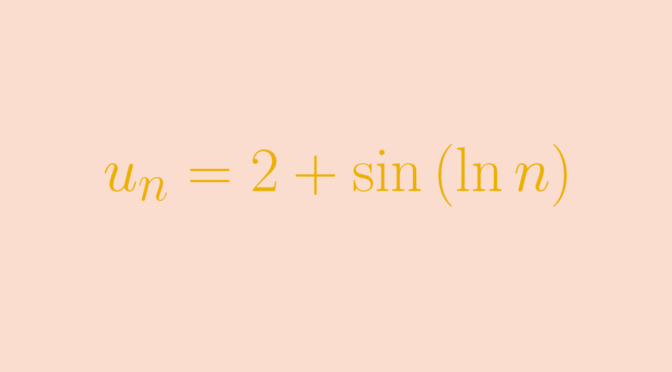Counterexamples on real sequences (part 3)

Let $$(u_n)$$ be a sequence of real numbers.

If $$u_{2n}-u_n \le \frac{1}{n}$$ then $$(u_n)$$ converges?

This is wrong. The sequence
$u_n=\begin{cases} 0 & \text{for } n \notin \{2^k \ ; \ k \in \mathbb N\}\\ 1- 2^{-k} & \text{for } n= 2^k\end{cases}$
is a counterexample. For $$n \gt 2$$ and $$n \notin \{2^k \ ; \ k \in \mathbb N\}$$ we also have $$2n \notin \{2^k \ ; \ k \in \mathbb N\}$$, hence $$u_{2n}-u_n=0$$. For $$n = 2^k$$ $0 \le u_{2^{k+1}}-u_{2^k}=2^{-k}-2^{-k-1} \le 2^{-k} = \frac{1}{n}$ and $$\lim\limits_{k \to \infty} u_{2^k} = 1$$. $$(u_n)$$ does not converge as $$0$$ and $$1$$ are limit points.

If $$\lim\limits_{n} \frac{u_{n+1}}{u_n} =1$$ then $$(u_n)$$ has a finite or infinite limit?

This is not true. Let’s consider the sequence
$u_n=2+\sin(\ln n)$ Using the inequality $$\vert \sin p – \sin q \vert \le \vert p – q \vert$$
which is a consequence of the mean value theorem, we get $\vert u_{n+1} – u_n \vert = \vert \sin(\ln (n+1)) – \sin(\ln n) \vert \le \vert \ln(n+1) – \ln(n) \vert$ Therefore $$\lim\limits_n \left(u_{n+1}-u_n \right) =0$$ as $$\lim\limits_n \left(\ln(n+1) – \ln(n)\right) = 0$$. And $$\lim\limits_{n} \frac{u_{n+1}}{u_n} =1$$ because $$u_n \ge 1$$ for all $$n \in \mathbb N$$.

I now assert that the interval $$[1,3]$$ is the set of limit points of $$(u_n)$$. For the proof, it is sufficient to prove that $$[-1,1]$$ is the set of limit points of the sequence $$v_n=\sin(\ln n)$$. For $$y \in [-1,1]$$, we can pickup $$x \in \mathbb R$$ such that $$\sin x =y$$. Let $$\epsilon > 0$$ and $$M \in \mathbb N$$ , we can find an integer $$N \ge M$$ such that $$0 < \ln(n+1) - \ln(n) \lt \epsilon$$ for $$n \ge N$$. Select $$k \in \mathbb N$$ with $$x +2k\pi \gt \ln N$$ and $$N_\epsilon$$ with $$\ln N_\epsilon \in (x +2k\pi, x +2k\pi + \epsilon)$$. This is possible as $$(\ln n)_{n \in \mathbb N}$$ is an increasing sequence and the length of the interval $$(x +2k\pi, x +2k\pi + \epsilon)$$ is equal to $$\epsilon$$. We finally get $\vert u_{N_\epsilon} - y \vert = \vert \sin \left(\ln N_\epsilon \right) - \sin \left(x + 2k \pi \right) \vert \le \left(\ln N_\epsilon - (x +2k\pi)\right) \le \epsilon$ proving that $$y$$ is a limit point of $$(u_n)$$.